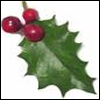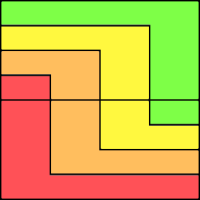#### You may also like### Giant Holly Leaf

Find the perimeter and area of a holly leaf that will not lie flat (it has negative curvature with 'circles' having circumference greater than 2πr).Given a square ABCD of sides 10 cm, and using the corners as centres, construct four quadrants with radius 10 cm each inside the square. The four arcs intersect at P, Q, R and S. Find the area enclosed by PQRS.### Get Cross

A white cross is placed symmetrically in a red disc with the central square of side length sqrt 2 and the arms of the cross of length 1 unit. What is the area of the disc still showing?

# Curvy Areas

### Why do this problem?

This problem offers the opportunity to practise calculating areas of semicircles and working in terms of $\pi$ and leads to a surprising result that invites students to generalise.

### Possible approach

These printable resources may be useful: Curvy Areas,
Curvy Areas.

Begin by showing one of the three diagrams on these slides for a short while, then hide it.
"Think about the image you just saw. Can you make a sketch of it? Can you describe to your partner how it was drawn?"

Show the image again so that students can compare their first impression with the actual image. Recreating the image offers students a good opportunity for practising constructions with a pair of compasses.

"Now that we can see how the images were created, talk to your partner and see if you can come up with a method for working out the shaded areas." Give students a little time to discuss, and then bring the class together to share their suggested methods. Then ask students to use these methods to work out all the areas for each curvy pattern.

Students might need prompting to choose a letter or value to represent the radius of the smallest semi-circle in order to work out the areas. There is scope for some discussion here about the merits of assigning a unit length rather than using a variable, and why this is 'allowed' in a question asking about proportion.

Surprisingly, each coloured region on a diagram is the same proportion of the total area. Once students have found that result, they could work on a pattern with more regions to see if the same result follows.

To prove the general case that all regions have equal area is quite challenging. A more accessible question to work on first of all is to show that the first region (coloured red in our diagrams) is always $\frac{1}{n}$ of the total area, for a shape with $n$ regions.

The diagram below builds a similar pattern from rectangles and could be used to develop appropriate arguments, without needing to include $\pi$ in calculations.### Key questions

Where are the centres of semicircles in the diagrams?
What is the relationship between the different radii?
For a circle with $n$ different coloured sections, how would you work out the red area?

### Possible support

The problem An Unusual Shape provides practice in calculating the areas of semicircles.
The problem Blue and White investigates sequences based on the areas of circles.

### Possible extension

Prove algebraically that all sections have equal area for a circle with $n$ sections